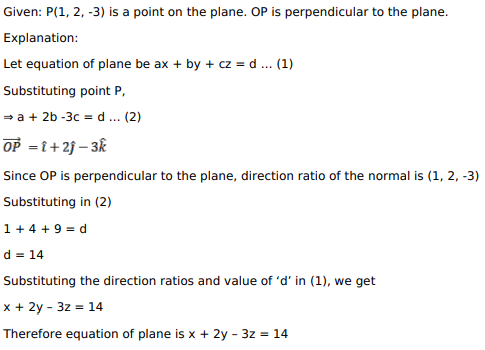# Mark against the correct answer in each of the following:

Question:

Mark against the correct answer in each of the following:

If $O$ is the origin and $P(1,2,-3)$ is a given point, then the equation of the plane through $P$ and perpendicular to OP is

A. $x+2 y-3 z=14$

B. $x-2 y+3 z=12$

C. $x-2 y-3 z=14$

D. None of these

Solution: